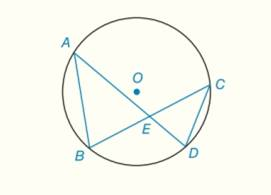Chapter 6.CT, Problem 16CT### Elementary Geometry for College St...

6th Edition
Daniel C. Alexander + 1 other
ISBN: 9781285195698

#### Solutions

Chapter
Section### Elementary Geometry for College St...

6th Edition
Daniel C. Alexander + 1 other
ISBN: 9781285195698
Textbook Problem
1 views

# Provide the missing statements and reasons in the following proof.Given: In ⊙ O , chords A D ¯   and B C ¯ intersects at E .Prove: A E C E = B E D EPROOF Statements Reasons 1.                                                               _ 1.                                                               _ 2. ∠ A E B ≅ ∠ D E C 2.                                                               _ 3. ∠ B ≅ ∠ D 3.If two inscribed angles intercept the same arc, these angles are congruent 4. Δ A B E ∼ Δ C D E 4.                                                               _ 5.                                                               _ 5.CSSTP

To determine

To prove:

AECE=BEDE by providing the missing statement in,

 PROOF Statements Reasons 1.                                _ 1.                                _ 2. ∠AEB≅∠DEC 2.                                _ 3. ∠B≅∠D 3.If two inscribed angles intercept the same arc, these angles are congruent 4. ΔABE∼ΔCDE 4.                                _ 5.                                _ 5.CSSTP
Explanation

Given:

In O, chords AD¯ and BC¯ intersects at E.

 PROOF Statements Reasons 1.                                _ 1.                                _ 2. ∠AEB≅∠DEC 2.                                _ 3. ∠B≅∠D 3

### Still sussing out bartleby?

Check out a sample textbook solution.

See a sample solution

#### The Solution to Your Study Problems

Bartleby provides explanations to thousands of textbook problems written by our experts, many with advanced degrees!

Get Started

#### 11. Is if?

Mathematical Applications for the Management, Life, and Social Sciences

#### In Exercises 516, evaluate the given quantity. log1,000

Finite Mathematics and Applied Calculus (MindTap Course List)

#### j × (−k) = i −i j + k −j − k

Study Guide for Stewart's Multivariable Calculus, 8th